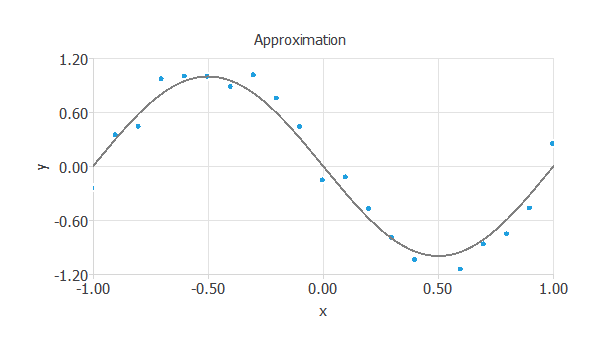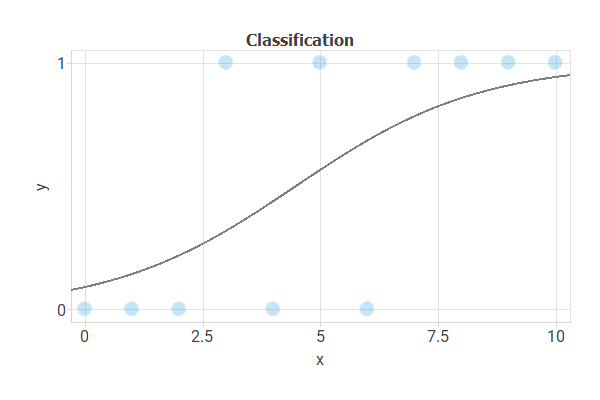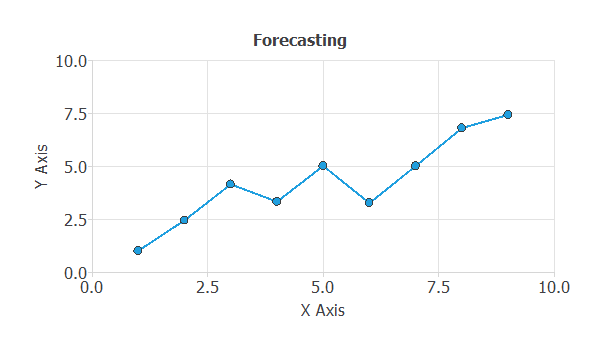﻿ Neural networks tutorial: Application types

1. Model types

Neural networks use information in the form of data to generate knowledge in the form of models.A model can be defined as a description of a real-world system or process using mathematical concepts. It is usually represented as a mapping between input and output variables.

In this regard, neural networks are used to discover relationships, recognize patterns, predict trends, and recognize associations from data.

Regarding their type, most neural network models belong to the following types:1.1. Approximation (or function regression)

An approximation can be regarded as the problem of fitting a function from data.

Here the neural network learns from knowledge represented by a data set consisting of instances with input and target variables. The targets are a specification of the response to the inputs.

In this regard, the basic goal in an approximation problem is to model one or several target variables, conditioned on the input variables.

The following figure illustrates an approximation problem. The objective here is to estimate the value of $y$, knowing the value of $x$.In approximation applications, the targets are usually continuous variables. Some examples are the following:

A common feature of most data sets is that the data exhibits an underlying systematic aspect, represented by some function, but is corrupted with random noise.

The objective is to produce a neural network which exhibits good generalization, or in other words, one which makes good predictions for new data.

The best generalization is obtained when the mapping represents the underlying systematic aspects of the data, rather than capturing the specific details (i.e., the noise contribution).1.2. Classification (or pattern recognition)

Classification can be stated as the process whereby a received pattern, characterized by a distinct set of features, is assigned to one of a prescribed number of classes.

The inputs here include a set of features that characterize a pattern; the targets specify the class that each pattern belongs to.

The basic goal in a classification problem is to model the posterior probabilities of class membership, conditioned on the input variables.

The next figure illustrates a classification task. Here, we want to discriminate the class (round circles or green squares) based on the $x_1$ and $x_2$ values of the object.As before, the central goal is to design a neural network with good generalization capabilities. That is a model that can classify new data correctly.

We can distinguish between two types of classification models:

Binary classification

In binary classification, the target variable is usually binary (true or false).

Some examples are to:

Multiple classification

In multiple classification, the target variable is usually nominal (class_1, class_2, or class_3).

Some examples are the following:1.3. Forecasting (or time series prediction)

Forecasting can be regarded as the problem of predicting the future state of a system from a set of past observations to that system.

A forecasting application can also be solved by approximating a function from a data set. In this type of problems, the data is arranged in a time series fashion. The inputs include data from past observations (predictors) and the targets include corresponding future observations (predictands).

The basic goal here is to model the future observations, conditioned on the past observations.

The following figure illustrates a forecast application. The objective is to predict a step ahead $y_{t+1}$, based on the lags $y_{t}, y_{t-1},...$.An example is to forecast the future sales of a car model, as a function of past sales, marketing actions and macroeconomic variables.

As always, good generalization is the central goal in forecasting applications.